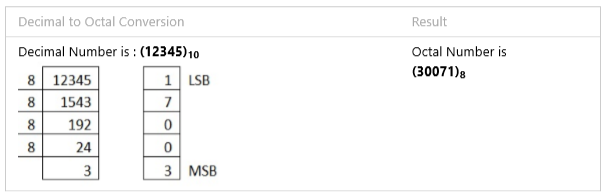Type Here to Get Search Results !

# Number system: Computer Application in Pharmacy Pharmacy

## Number system: Binary number system, Decimal number system, Octal number system, Hexadecimal number systems, conversion decimal to binary, binary to decimal, octal to binary etc, binary addition, binary subtraction – One’s complement ,Two’s complement method, binary multiplication, binary division

The technique to represent and work with numbers is called number system. Decimal number system is the most common number system. Other popular number systems include binary number system, octal number system, hexadecimal number system, etc.

### Decimal Number System

Decimal number system is a base 10 number system having 10 digits from 0 to 9. This means that any numerical quantity can be represented using these 10 digits. Decimal number system is also a positional value system. This means that the value of digits will depend on its position. Let us take an example to understand this.
In digital systems, instructions are given through electric signals; variation is done by varying the voltage of the signal. Having 10 different voltages to implement decimal number system in digital equipment is difficult. So, many number systems that are easier to implement digitally have been developed. Let’s look at them in detail.

### Binary Number System

The easiest way to vary instructions through electric signals is two-state system – on and off. On is represented as 1 and off as 0, though 0 is not actually no signal but signal at a lower voltage. The number system having just these two digits – 0 and 1 – is called binary number system.
Each binary digit is also called a bit. Binary number system is also positional value system, where each digit has a value expressed in powers of 2, as displayed here.
In any binary number, the rightmost digit is called least significant bit  and leftmost digit is called most significant bit .
And decimal equivalent of this number is sum of product of each digit with its positional value.
Computer memory is measured in terms of how many bits it can store. Here is a chart for memory capacity conversion.
• 1 byte  = 8 bits
• 1 Kilobytes  = 1024 bytes
• 1 Megabyte  = 1024 KB
• 1 Gigabyte  = 1024 MB
• 1 Terabyte  = 1024 GB
• 1 Exabyte  = 1024 PB
• 1 Zettabyte = 1024 EB
• 1 Yottabyte  = 1024 ZB

### Octal Number System

Octal number system has eight digits – 0, 1, 2, 3, 4, 5, 6 and 7. Octal number system is also a positional value system with where each digit has its value expressed in powers of 8, as shown here −
Decimal equivalent of any octal number is sum of product of each digit with its positional value.

Octal number system has 16 symbols – 0 to 9 and A to F where A is equal to 10, B is equal to 11 and so on till F. Hexadecimal number system is also a positional value system with where each digit has its value expressed in powers of 16, as shown here
Decimal equivalent of any hexadecimal number is sum of product of each digit with its positional value.

### Number System Conversions

There are three types of conversion:
• Decimal Number System to Other Base [ for example: Decimal Number System to Binary Number System]
• Other Base to Decimal Number System [ for example: Binary Number System to Decimal Number System]
• Other Base to Other Base [ for example: Binary Number System to Hexadecimal Number System]

### Decimal Number System to Other Base

To convert Number system from Decimal Number System to Any Other Base is quite easy; you have to follow just two steps:
1. Divide the Number (Decimal Number) by the base of target base system (in which you want to convert the number: Binary (2), octal (8) and Hexadecimal (16)).
2. Write the remainder from step 1 as a Least Signification Bit (LSB) to Step last as a Most Significant Bit (MSB).\### Other Base System to Decimal Number Base

To convert Number System from Any Other Base System to Decimal Number System, you have to follow just three steps:
1. Determine the base value of source Number System (that you want to convert), and also determine the position of digits from LSB (first digit’s position – 0, second digit’s position – 1 and so on).
2. Multiply each digit with its corresponding multiplication of position value and Base of Source Number System’s Base.
3. Add the resulted value in step-B.
Explanation regarding examples:
1. Below given exams contains the following rows:
2. Row 1 contains the DIGITs of number (that is going to be converted).
3. Row 2 contains the POSITION of each digit in the number system.
4. Row 3 contains the multiplication: DIGIT* BASE^POSITION
5. Row 4 contains the calculated result of step C.
6. And then add each value of step D, resulted value is the Decimal Number.

1.